# Flatten Binary Tree to Linked List

Problem Statement: Flatten Binary Tree To Linked List. Write a program that flattens a given binary tree to a linked list.

Note:

• The sequence of nodes in the linked list should be the same as that of the preorder traversal of the binary tree.
• The linked list nodes are the same binary tree nodes. You are not allowed to create extra nodes.
• The right child of a node points to the next node of the linked list whereas the left child points to NULL.
```Example: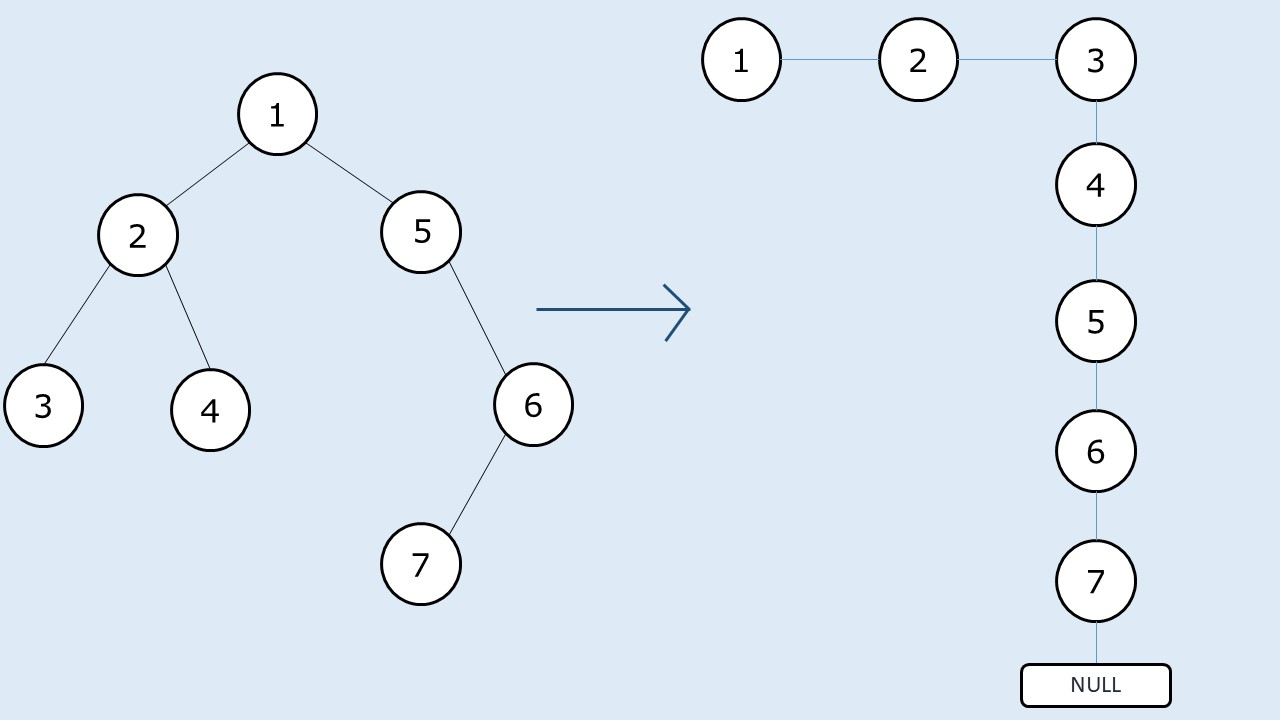```

Pre-req: Traversal Techniques

Disclaimer: Don’t jump directly to the solution, try it out yourself first.

### Solution 1: Using Recursion

Intuition:

The sequence of nodes in the linked list should be that of the preorder traversal. We will take a smaller example.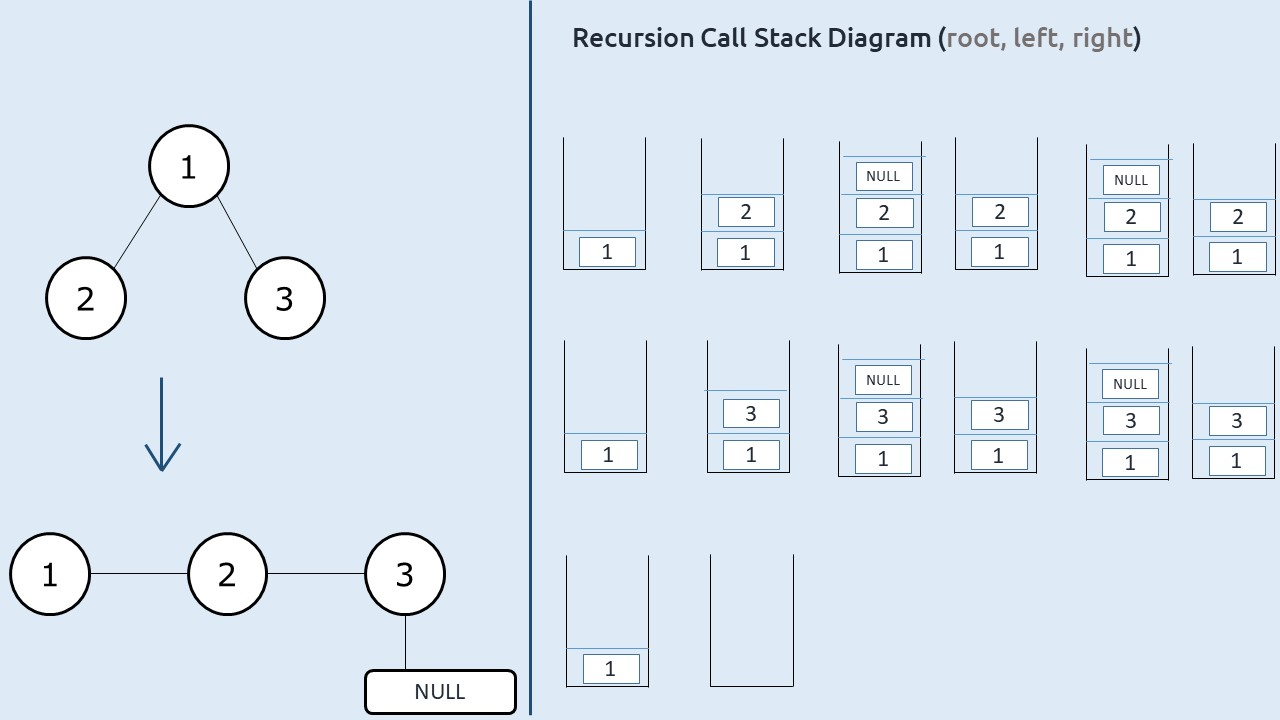At starting node 1, what we finally want is that node 1’s right child should point to its current left child (node 2). Now If we set it like this in our preorder traversal there will be no way to reach node 3.

We need to modify our traversal technique. If we somehow start at node 3 (last node of the linked list), we need not traverse its right child as it is NULL, therefore we can straightaway set its right child to its left child( which is again NULL) and set its left child to NULL. Now we need to get to the second last node of the linked list (node 2) and set the right child to node 3. After that, we need to move to the third last node i.e node 1.

Approach:

The algorithm steps can be stated as:

• If we observe, we are moving in a reverse postorder way : i.e  right, left, root.
• We take a reference variable (say prev) to store the previous node( initialized to NULL).
• Whenever we visit a node, we set the right child to the prev and left child to NULL.
• Next we assign this current node to prev.
• We perform the above two operations on all the nodes in the traversal.

Dry run:

The following illustrations will give a clear idea.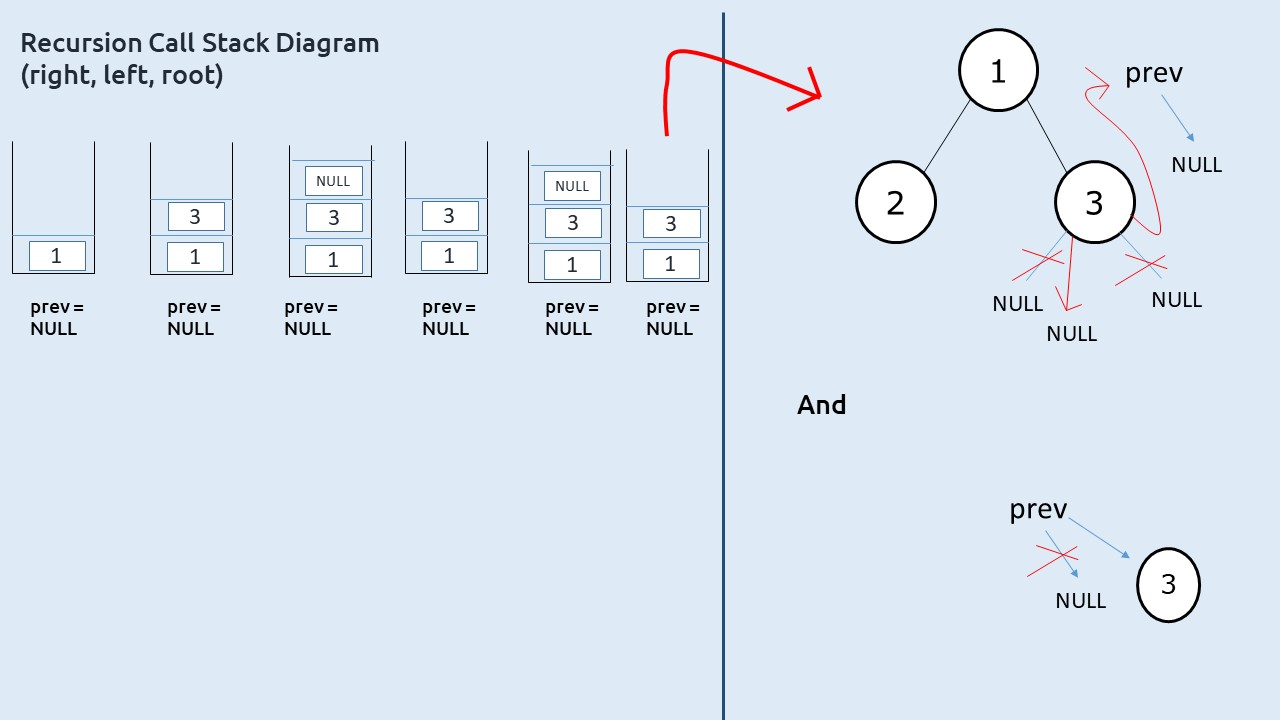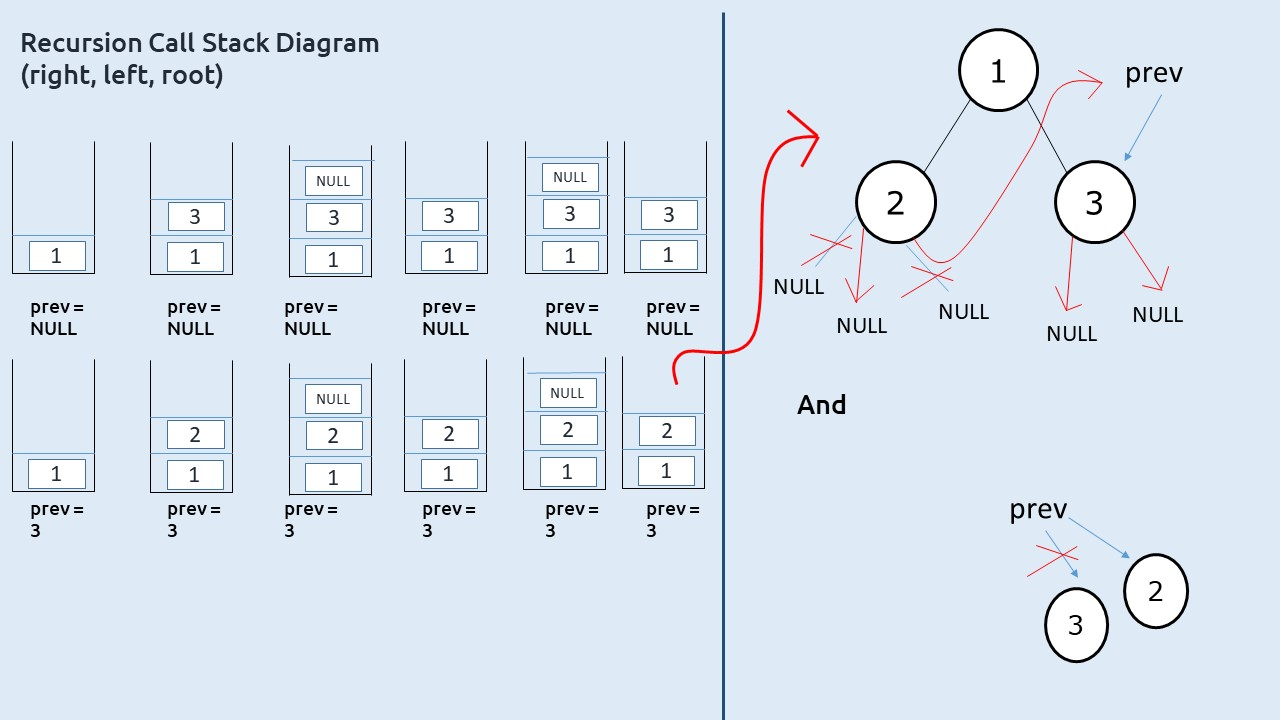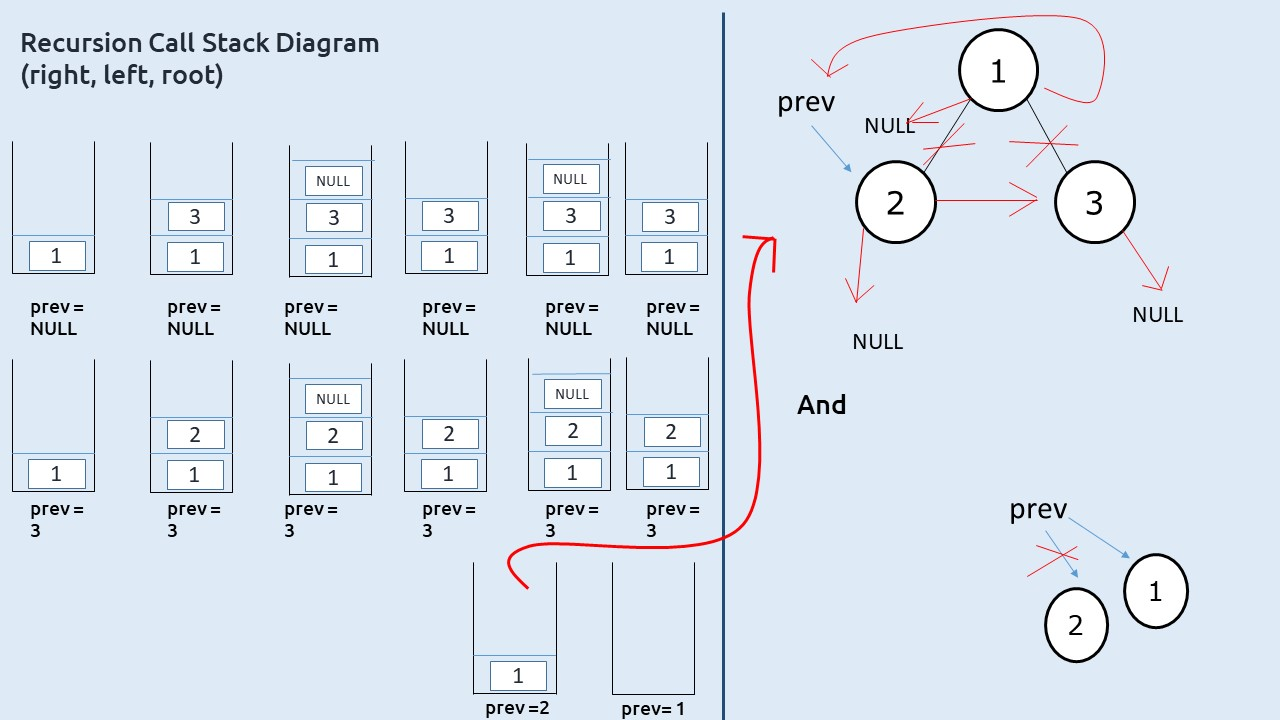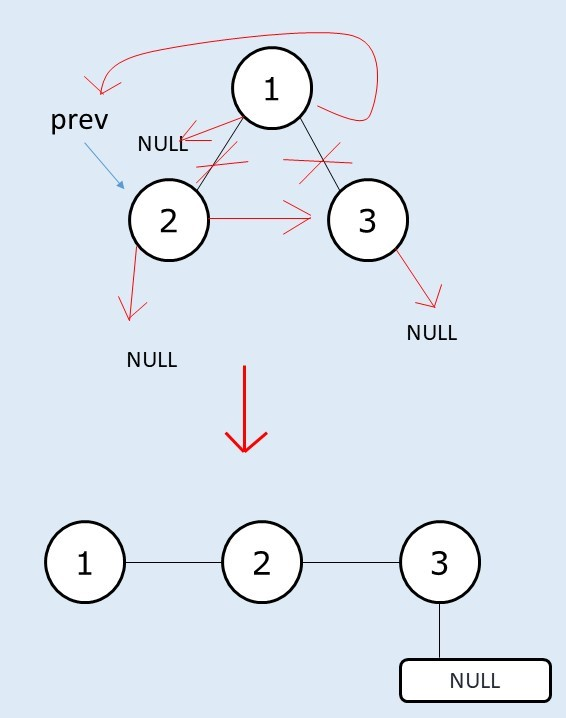Code:

## C++ Code

``````#include <bits/stdc++.h>

using namespace std;

struct node {
int data;
struct node * left, * right;
};

class Solution {
node * prev = NULL;
public:
void flatten(node * root) {
if (root == NULL) return;

flatten(root -> right);
flatten(root -> left);

root -> right = prev;
root -> left = NULL;
prev = root;
}

};

struct node * newNode(int data) {
struct node * node = (struct node * ) malloc(sizeof(struct node));
node -> data = data;
node -> left = NULL;
node -> right = NULL;

return (node);
}

int main() {

struct node * root = newNode(1);
root -> left = newNode(2);
root -> left -> left = newNode(3);
root -> left -> right = newNode(4);
root -> right = newNode(5);
root -> right -> right = newNode(6);
root -> right -> right -> left = newNode(7);

Solution obj;

obj.flatten(root);
while(root->right!=NULL)
{
cout<<root->data<<"->";
root=root->right;
}
cout<<root->data;
return 0;
}
``````

Output:

1->2->3->4->5->6->7

Time Complexity: O(N)

Reason: We are doing a simple preorder traversal.

Space Complexity: O(N)

Reason: Worst case time complexity will be O(N) (in case of skewed tree).

## Java Code

``````import java.util.*;

class Node {
int data;
Node  left, right;
Node(int data)
{
this.data=data;
left=null;
right=null;
}
}

public class Solution {
static Node prev = null;
static void flatten(Node  root) {
if (root == null) return;

flatten(root . right);
flatten(root . left);

root . right = prev;
root . left = null;
prev = root;
}

public static void main(String args[]) {

Node  root = new Node(1);
root . left = new Node(2);
root . left . left = new Node(3);
root . left . right = new Node(4);
root . right = new Node(5);
root . right . right = new Node(6);
root . right . right . left = new Node(7);
flatten(root);
while(root.right!=null)
{
System.out.print(root.data+"->");
root=root.right;
}
System.out.print(root.data);
}
}
``````

Output:

1->2->3->4->5->6->7

Time Complexity: O(N)

Reason: We are doing a simple preorder traversal.

Space Complexity: O(N)

Reason: Worst case time complexity will be O(N) (in case of skewed tree).

Solution 2: Iterative Approach – Using Stack

Intuition:

In a binary tree, generally, we need to set the right child of the node to the left and the left child to NULL. If the given tree is a left-skewed tree, this action alone will flatten the binary tree.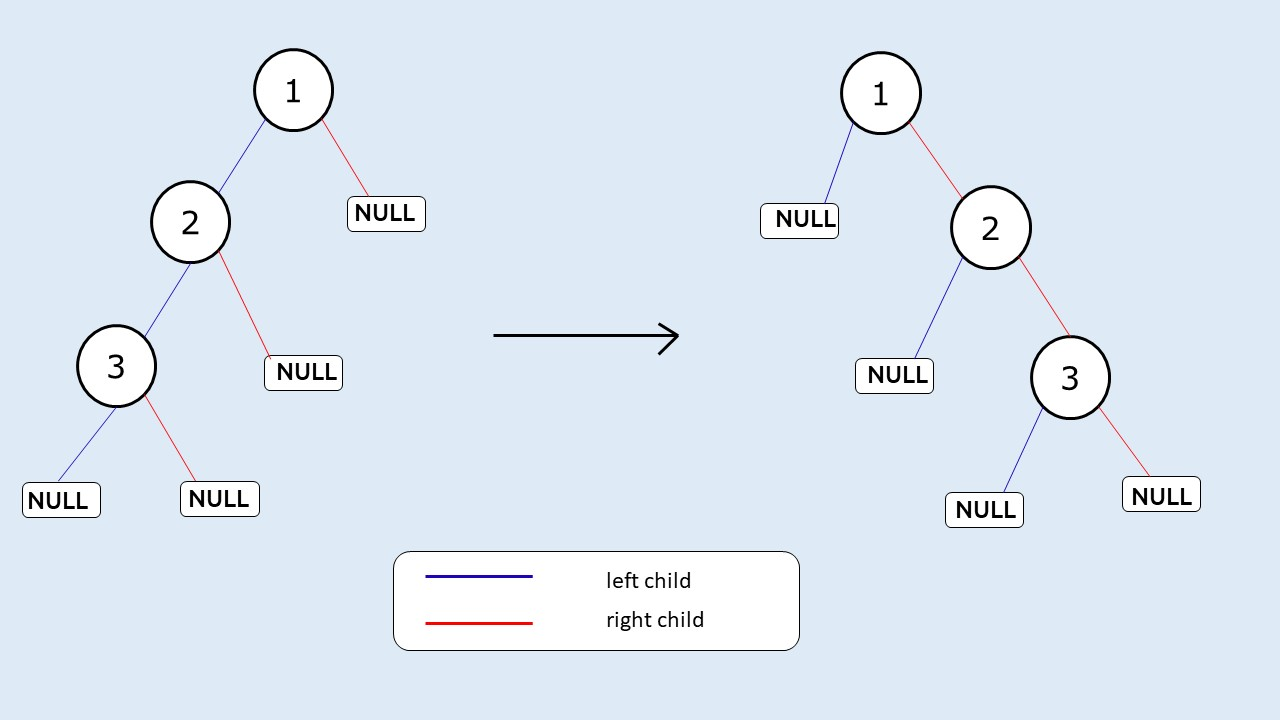Now the main question arises is what if the current node doesn’t have a left child? In that case, we don’t want to assign its right child to NULL( its left child), rather we want it to assign to itself so that our preorder sequence is maintained. In case the right child is also not present(a leaf node) we would want to assign the right child to some parent node’s right child.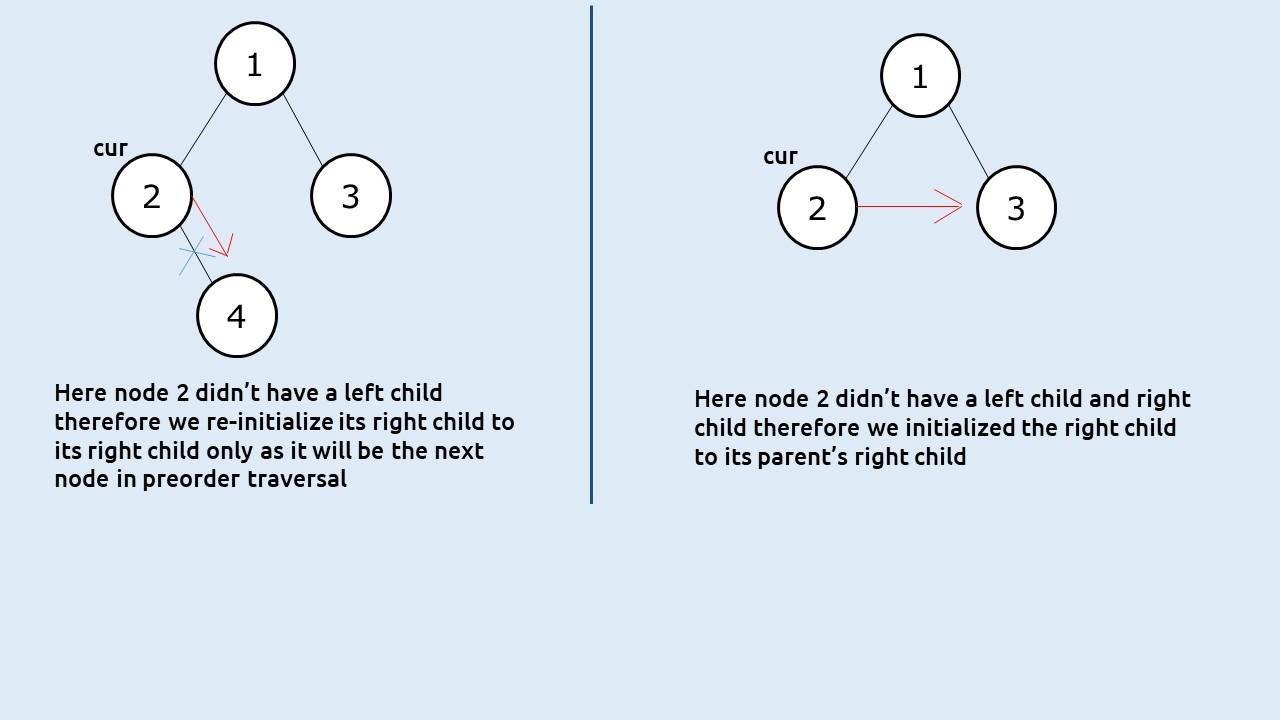To get to this parent’s right node we will use a stack. Whenever we are at a node we want to prioritize its left child if it is present. If it is not present we want to look at the right child. A stack is a LIFO data structure, we first push the right child and then the left child. Then we set the right child of the node to the stack’s top and left child as NULL. This way the stack always provides the correct next node.

Approach:

The algorithm approach can be stated as:

• Take a stack and push the root node to it.
• Set a while loop till the stack is non-empty.
• In every iteration, take the node at the top of the stack( say cur) and pop the stack.
• If cur has a right child, push it to the stack.
• If cur has a left child, push it to the stack.
• Set the right child of cur to node at stack’s top.
• Set the left child of cur as NULL.

Dry Run:

We will take a smaller example.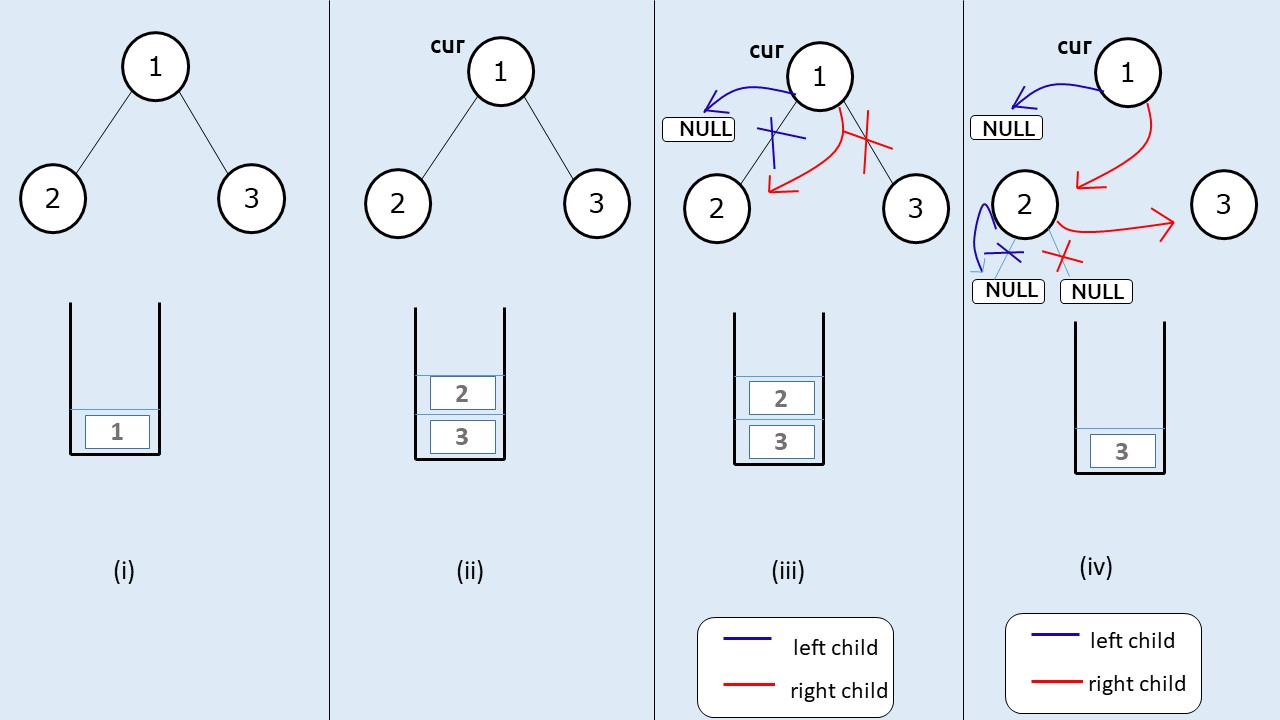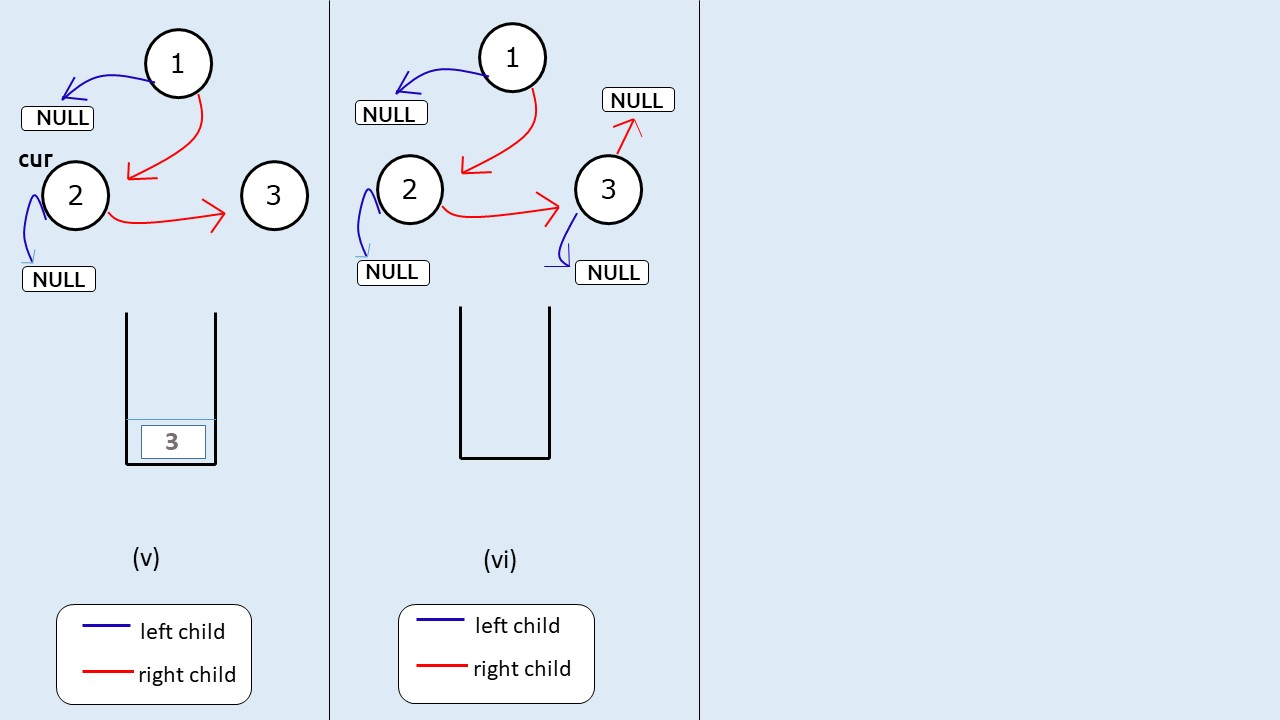Code:

## C++ Code

``````#include <bits/stdc++.h>

using namespace std;

struct node {
int data;
struct node * left, * right;
};

class Solution {
node * prev = NULL;
public:
void flatten(node * root) {
if (root == NULL) return;
stack < node * > st;
st.push(root);
while (!st.empty()) {
node * cur = st.top();
st.pop();

if (cur -> right != NULL) {
st.push(cur -> right);
}
if (cur -> left != NULL) {
st.push(cur -> left);
}
if (!st.empty()) {
cur -> right = st.top();
}
cur -> left = NULL;
}

}

};

struct node * newNode(int data) {
struct node * node = (struct node * ) malloc(sizeof(struct node));
node -> data = data;
node -> left = NULL;
node -> right = NULL;

return (node);
}

int main() {

struct node * root = newNode(1);
root -> left = newNode(2);
root -> left -> left = newNode(3);
root -> left -> right = newNode(4);
root -> right = newNode(5);
root -> right -> right = newNode(6);
root -> right -> right -> left = newNode(7);

Solution obj;

obj.flatten(root);
while(root->right!=NULL)
{
cout<<root->data<<"->";
root=root->right;
}
cout<<root->data;
return 0;
}
``````

Output:

1->2->3->4->5->6->7

Time Complexity: O(N)

Reason: The loop will execute for every node once.

Space Complexity: O(N)

## Java Code

``````import java.util.*;

class Node {
int data;
Node  left, right;
Node(int data)
{
this.data=data;
left=null;
right=null;
}
}

public class Solution {
static Node prev = null;
static void flatten(Node  root) {
if (root == null) return;
Stack < Node  > st=new Stack<>();
st.push(root);
while (!st.isEmpty()) {
Node  cur = st.peek();
st.pop();

if (cur . right != null) {
st.push(cur . right);
}
if (cur . left != null) {
st.push(cur . left);
}
if (!st.isEmpty()) {
cur . right = st.peek();
}
cur . left = null;
}

}

public static void main(String args[]) {

Node  root = new Node(1);
root . left = new Node(2);
root . left . left = new Node(3);
root . left . right = new Node(4);
root . right = new Node(5);
root . right . right = new Node(6);
root . right . right . left = new Node(7);
flatten(root);
while(root.right!=null)
{
System.out.print(root.data+"->");
root=root.right;
}
System.out.print(root.data);
}
}
``````

Output:

1->2->3->4->5->6->7

Time Complexity: O(N)

Reason: The loop will execute for every node once.

Space Complexity: O(N)

Solution 3 – Using Intuition behind Morris Traversal.

Pre- req: Morris Traversal

Intuition: We will use the intuition behind morris’s traversal. In Morris Traversal we use the concept of a threaded binary tree.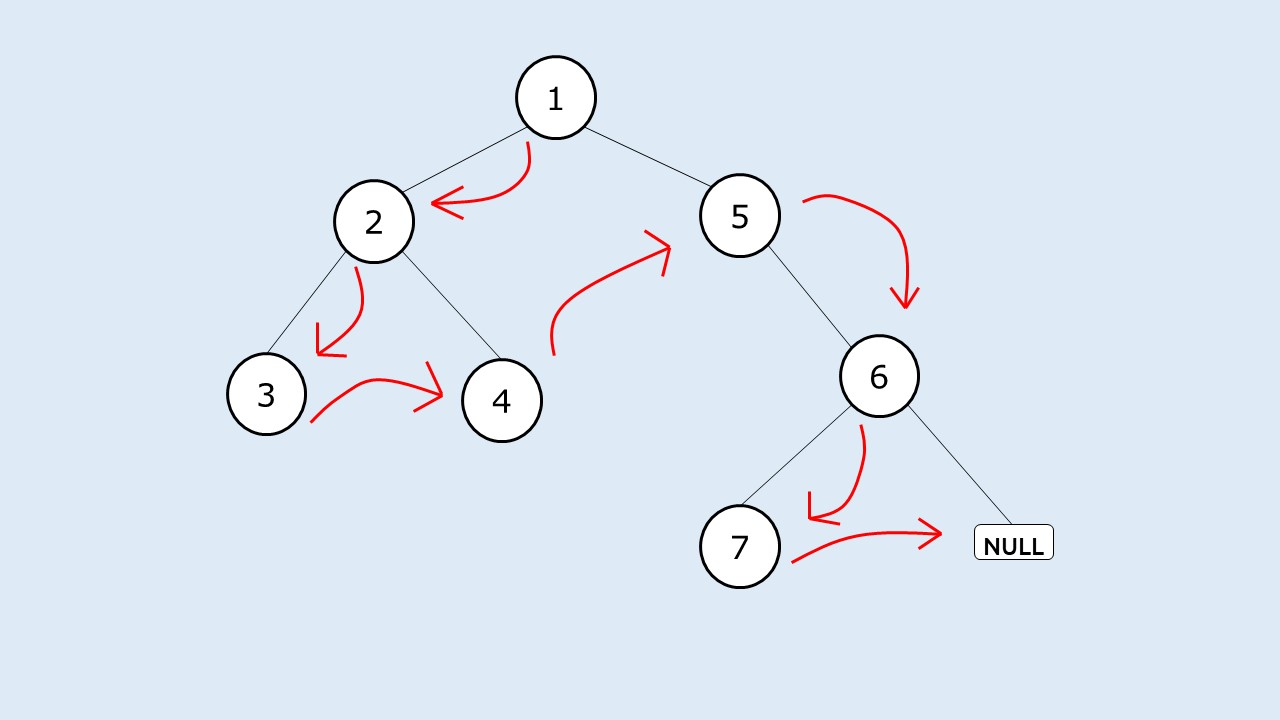If we set the right child of every node like this(marked in red) and the left child as NULL, our job will be done.

Approach:

The algorithm can be described as:

• At a node(say cur) if there exists a left child, we will find the rightmost node in the left subtree(say prev).
• We will set prev’s right child to cur’s right child,
• We will then set cur’s right child to it’s left child.
• We will then move cur to the next node by assigning cur it to its right child
• We will stop the execution when cur points to NULL.

Dry Run:

We will take a smaller example.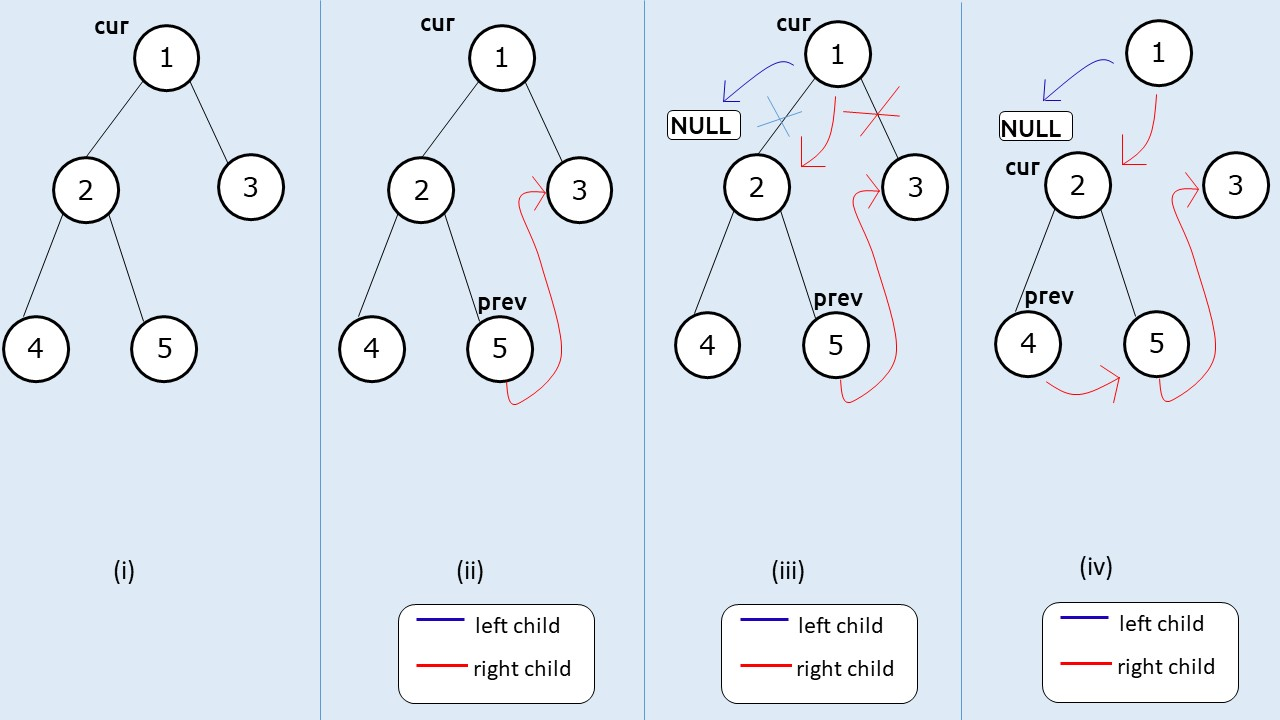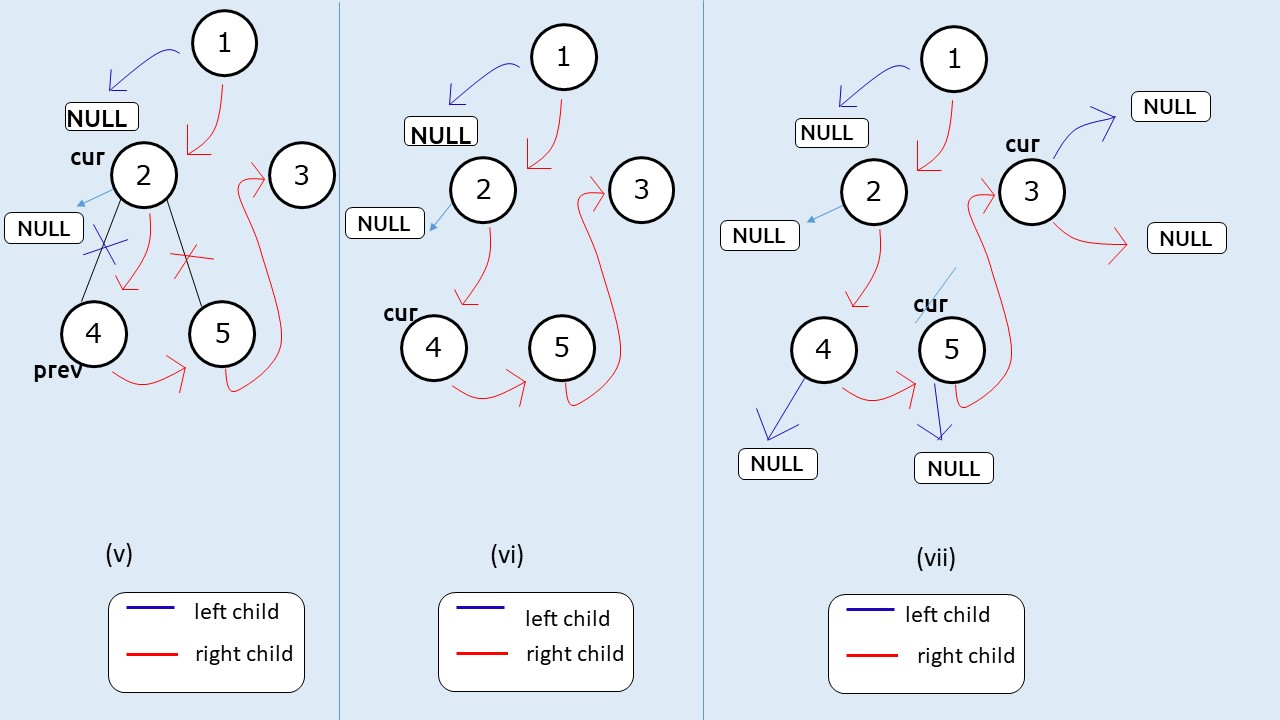Code:

## C++ Code

``````#include <bits/stdc++.h>

using namespace std;

struct node {
int data;
struct node * left, * right;
};

class Solution {
node * prev = NULL;
public:
void flatten(node* root) {
node* cur = root;
while (cur)
{
if(cur->left)
{
node* pre = cur->left;
while(pre->right)
{
pre = pre->right;
}
pre->right = cur->right;
cur->right = cur->left;
cur->left = NULL;
}
cur = cur->right;
}
}

};

struct node * newNode(int data) {
struct node * node = (struct node * ) malloc(sizeof(struct node));
node -> data = data;
node -> left = NULL;
node -> right = NULL;

return (node);
}

int main() {

struct node * root = newNode(1);
root -> left = newNode(2);
root -> left -> left = newNode(3);
root -> left -> right = newNode(4);
root -> right = newNode(5);
root -> right -> right = newNode(6);
root -> right -> right -> left = newNode(7);

Solution obj;

obj.flatten(root);
while(root->right!=NULL)
{
cout<<root->data<<"->";
root=root->right;
}
cout<<root->data;
return 0;
}
``````

Output:

1->2->3->4->5->6->7

Time Complexity: O(N)

Reason: Time complexity will be the same as that of a morris traversal

Space Complexity: O(1)

Reason: We are not using any extra space.

## Java Code

``````import java.util.*;

class Node {
int data;
Node  left, right;
Node(int data)
{
this.data=data;
left=null;
right=null;
}
}

public class Solution {
static Node prev = null;
static void flatten(Node root) {
Node cur = root;
while (cur!=null)
{
if(cur.left!=null)
{
Node pre = cur.left;
while(pre.right!=null)
{
pre = pre.right;
}
pre.right = cur.right;
cur.right = cur.left;
cur.left = null;
}
cur = cur.right;
}
}

public static void main(String args[]) {

Node  root = new Node(1);
root . left = new Node(2);
root . left . left = new Node(3);
root . left . right = new Node(4);
root . right = new Node(5);
root . right . right = new Node(6);
root . right . right . left = new Node(7);
flatten(root);
while(root.right!=null)
{
System.out.print(root.data+"->");
root=root.right;
}
System.out.print(root.data);
}
}
``````

Output:

1->2->3->4->5->6->7

Time Complexity: O(N)

Reason: Time complexity will be the same as that of a morris traversal

Space Complexity: O(1)

Reason: We are not using any extra space.

Special thanks to Anshuman Sharma for contributing to this article on takeUforward. If you also wish to share your knowledge with the takeUforward fam, please check out this article